# Chapter XV The Lengths of Curves

## 162 General formula for the length of a curve in Cartesian co-ordinates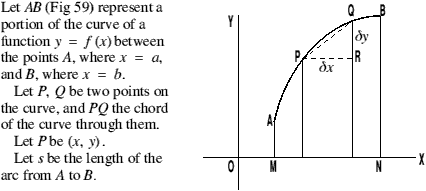Figure 59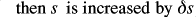i.e.represents the length of the arc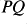.

Then by geometry the chord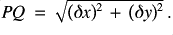If Q be taken close to P, i.e.becomes small, the length of the chord is nearly equal to the length of the arc.

If Q is indefinitely close to P, in the limit when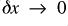, the chord approaches to coincidence when the curve and the sum of these chords is equal with the length of the arc.If the integration is more conveniently performed with respect to values of y, then, where c and d are limits of y.

In many cases the evaluation of the integral is difficult and requires a more advanced knowledge of the subject than is contained in this volume.

(Teach yourself calculus)